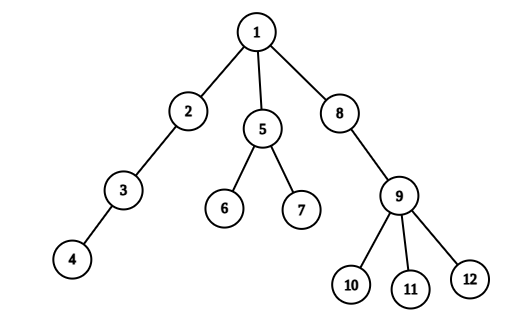0

# Tree Queries

Difficulty: NINJAContributed By
Arindam Majumder
Avg. time to solve
90 min
Success Rate
20%

Problem Statement

#### You are given a tree with 'N' vertices, where each vertex has an assigned integer. You are given 'Q' queries where each query can be of two types:

``````1 'U' 'V': Reverse the order of all the integers on the path between 'U' and 'V'.
2 'U' 'V': Print the sum of all the integers on the path between 'U' and 'V'.
``````
##### Input Format:
``````The first line contains two space-separated integers, ‘N’ and ‘Q’, denoting the number of vertices of the tree and the number of queries.

The next N-1 lines will contain two space-separated integers u and v, denoting an undirected edge between city u and city v.

The next line contains ‘N’ space-separated integers denoting the value associated with each node.

The next q lines contain three space-separated integers representing the queries of both types.
``````
##### Output Format:
``````Output for each query of type two only, whether the path between the two cities of that query is alternating or not.

Output for each query will be printed in a separate line.
``````
##### Note
``````You are not required to print anything; it has already been taken care of. Just implement the function.
``````
##### Constraints:
``````1 <= N,Q <= 10^4
1 <= u,v <= N
1 <= value[i] <= 10^4

Where value[i] represents the value associated with the node.

Time Limit: 1 sec.
``````
##### Sample Input 1:
``````12 3
1 2
2 3
3 4
1 5
5 6
5 7
1 8
8 9
9 10
9 11
9 12
10 8 5 9 12 16 8 18 21 11 19 20
2 4 7
1 1 6
2 4 7
``````
##### Sample Output 1:
``````52
58
``````
##### Explanation For Sample Output 1:``````In the first query, we have to find the sum of the values of the path between node 4 and node 7. The path between 4 and 7 is 4, 3, 2, 1, 5, 7, and their corresponding height is 9, 5, 8, 10, 12, 8.
Their sum is 52. So the output is 52.

In the second query, we have to reverse the order of the values on the path between 1 and 6. The path is 1 5 6, and their corresponding values are 10 12 16. After reversing, their values will be 16 12 10. So, the final array will be 16 8 5 9 12 10 8 18 21 11 19 20.

In the third query, we have to find the sum of the values of the path between node 4 and node 7. The path between 4 and 7 is 4, 3, 2, 1, 5, 7, and their corresponding height is 9, 5, 8, 16, 12, 8.
Their sum is 58. So the output is 58.
``````
##### Sample Input 2:
``````6 4
1 5
2 1
3 2
4 3
4 6
2 5 4 1 6 3
2 3 3
1 2 6
2 5 6
2 5 1
``````
##### Sample Output 2:
``````4
21
8
``````Console